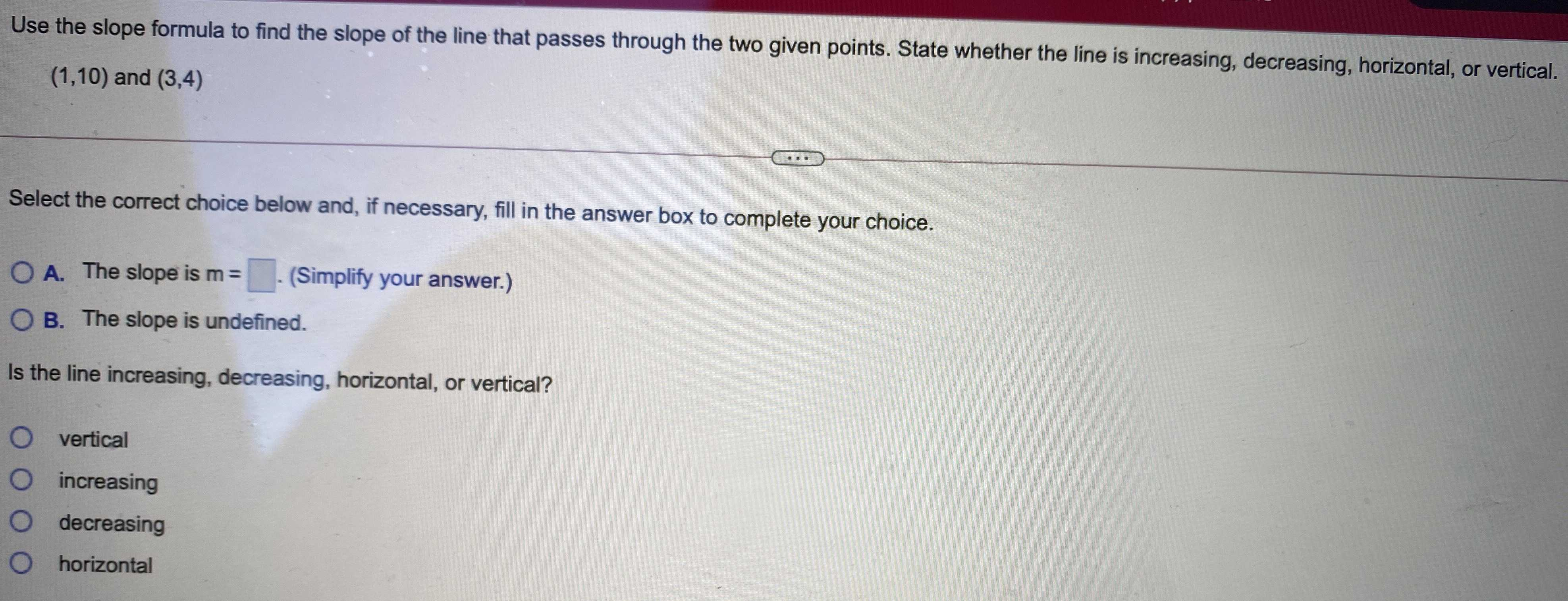### Still have math questions?

Algebra
QuestionUse the slope formula to find the slope of the line that passes through the two given points. State whether the line is increasing, decreasing, horizontal, or vertical.

$$( 1,10 )$$ and $$( 3,4 )$$

Select the correct choice below and, if necessary, fill in the answer box to complete your choice.

A. The slope is moct the correct choice below and, if necessary, fill in the answer box to complete your choice.

B. The slope is undefined. Is the line increasing, decreasing, horizontal, or vertical? vertical increasing decreasing horizontal.

Is the line increasing, decreasing, horizontal, or vertical?